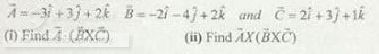# CSS Paper Physics 2017 Paper Part-II by FPSC Prepare Now

CSS Paper Physics 2017 Paper Part-II by FPSC Prepare Now

FEDERAL PUBLIC SERVICE COMMISSION
COMPETITIVE EXAMINATION – 2017
FOR RECRUITMENT TO POST IN BS – 17
UNDER THE FEDERAL GOVERNMENT

PHYSICS, PAPER – I

TIME ALLOWED: THREE HOURS
PART – I (MCQs): MAXIMUM 30 MINUTES
PART – I (MCQs): MAXIMUM MARKS = 20
PART – II MAXIMUM MARKS = 80

NOTE:
(i) Part – II is to be attempted on the separate Answer Book.
(ii) Attempt ONLY FOUR questions from PART – II. ALL questions carry EQUAL marks.
(iii) All the parts (if any) of each Question must be attempted at one place instead of at different places.
(iv) Candidate must write Q. No. in the Answer Book in Accordance with Q. No. in the Q. Paper.
(v) No Page / Space be left blank between the answers. All the blank pages of Answer Book must be crossed.
(iv) Extra attempt of any question or any part of the attempted question will not be considered.

PART – II

Q.2 (a) What is the cross product of two vectors? Why the cross product is called pseudo vector? (5)

(b) What is divergence of vector field? What is its physical significance? (5)

(c) What is line integral? Under what condition it is used to calculate the work done? (5)

(d) Consider three vector: (5)*****************************

Q.3 (a) What do you mean by circular motion? What is centrifugal force? Explain your answer by taking an example from daily life. (5)

(b) What is projectile motion? Why a cricket player lowers his hand while catching a ball? (7)

(c) What do you mean by work done by the system and work done on the system? Explain by talking a example of each. (5)

(d) A batsman hits a cricket ball at an angle with respect to the horizontal. The ball would strike the ground at 60m from the batsman if it is not stopped. But a fielder at a distance 55m catches the ball at a height of 1.5m. Calculate the angle of projection and the velocity of projection. (3)

*****************************

Q.4 (a) What do you mean by phase and group velocity? Derive a relation between a group and phase velocity. (7)

(b) What is superposition of waves? Show that the standing waves are produced by the superposition of two waves of equal amplitude moving in opposite direction. (7)

(c) A medium is disturbed by an oscillation described by,
Y=30cm sin(πx/10cm)cos(50πt)
Determine the amplitude，frequency，wavelength，speed，and direction of the component waves whose superposition produces this result. (3)

(d) If light of λ = 660nm has wave train λ20, what is its coherence length and coherence time? (3)

*****************************

Q. 5 (a) What is unique light from a laser source, and why should you never look directly into a laser beam? Explain briefly. (5)

(b) What is plasma? What do you mean by plasma frequency? Briefly discuss. (5)

(c) How the blue laser is useful in storing large amount of data on a CD as compared to red laser? (5)

(d) For the He-Ne laser at 2m and 4m distances from the laser, the output beam spot diameters are 2 mm and 3 mm. Calculate the angle of divergence. (5)

*****************************

Q.6 (a) What is viscosity? Discuss effect of temperature on the viscosity of liquids and gases. (6)

(b) Differentiate between streamline and turbulent flow and establish equation of continuity. (4)

(c) Explain why the level of mercury is down in capillary when placed in container of mercury, while it is up in the capillary in case of water? (6)

(d) A garden hose has an inside diameter of 2 cm and water flows through it is at 3 m/s.
(i) What nozzle diameter is required for the water to emerge at 10 m/s?
(ii) At what rate does the water leave the nozzle?

*****************************

Q.7 (a) What do you understand by classical statistical mechanics and quantum statistical mechanics? (6)

(b) Differentiate between Fermi-Dirac, Bose-Einstein and Maxwell-Boltzmann’s statistics. (6)

(c) What is equipartition of energy? Explain. (5)

(d) A 0.5m3 vessel is filled with air at atmospheric pressure. The air is churned by a paddle wheel attached to a shaft 0.1 m in diameter, rotating at a speed of 1800 rpm. A force of 5.0N acts on the rim of the shaft. What would be the pressure in the vessel after 10 seconds of operation? (3)

*****************************

Q.8 Write notes on any FOUR of the following: (5 each) (20)
(a) Polarization of light and its application in determining specific rotation of a liquid.
(b) Wave equation on a string
(c) Normal and anomalous dispersion of light
(d) Kinetic theory of gases
(e) Scalar Triple product

CSS Paper Physics 2017 Paper Part-II by FPSC Prepare Now